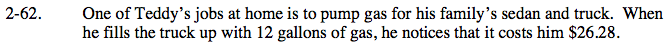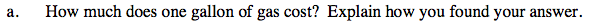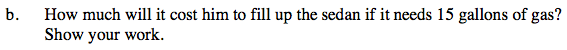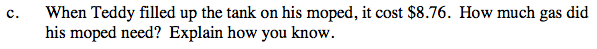### Home > CAAC > Chapter 2 > Lesson 2.1.6 > Problem2-62

2-62.$\frac{26.28}{12\text{ gals}}=\frac{n}{1\text{ gal}}$

Now multiply both sides by 12 gallons and solve.

$\frac{26.28}{12 \text{ gals}} \cdot 12 \text{ gals}=\frac{n}{1 \text{ gal}}\cdot 12\text{ gals}$

26.28 = 12n

$\frac{26.28}{12}=\frac{12n}{12}$

n = 2.19Multiply the price per gallon by the number of gallons.Use a proportion to show the known price (\$8.76) over the unknown number of gallons and follow the strategy shown in part (a) to solve.

$\frac{8.76}{\textit{n}}=\frac{2.19}{1}$